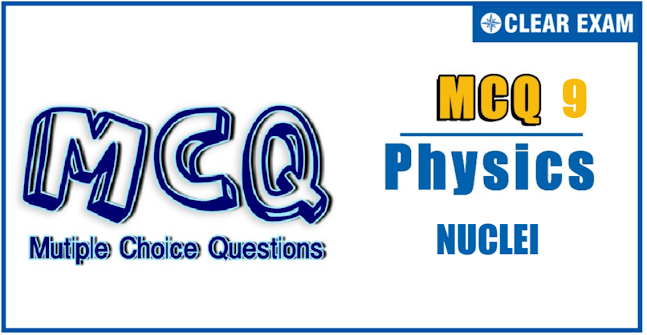## NUCLEI QUIZ-9

JEE Advanced Physics Syllabus can be referred by the IIT aspirants to get a detailed list of all topics that are important in cracking the entrance examination. JEE Advanced syllabus for Physics has been designed in such a way that it offers very practical and application-based learning to further make it easier for students to understand every concept or topic by correlating it with day-to-day experiences. In comparison to the other two subjects, the syllabus of JEE Advanced for physics is developed in such a way so as to test the deep understanding and application of concepts.

Q1In the fusion reaction _1^2 He+ _1^2 H→ _2^3 He+ _0^1 n, the masses of deuteron, helium and neutron expressed in a.m.u. are 2.015, 3.017 and 1.009, respectively. If 1 kg of deuterium undergoes complete fusion, find the amount of total energy released (1 a.m.u.=931.5 meV/c^2)
•   ≈6.02×10^13 J
•   ≈5.6×10^13 J
•   ≈9.0×10^13 J
•   ≈0.9×10^13 J
Q2. Uranium ores contain one radium-226 atom for every 2.8×10^6 uranium-238 atoms. Calculate the half-life of _92 U^238, given that the half-life of _88 Ra^226 is 1600 years (_88 Ra^226 is a decay product of _92 U^238)
•   1.75×10^3 years
•   1600×238/92 years
•   4.5×10^9 years
•   1600×238 years
Q3 Atomic masses of two isobars _29^64 Cu and _30^64 Zn are 63.9298 u and 63.9292 u, respectively. It can be concluded from this data that
•   Both the isobars are stable
•   _ ^64 Zn is radioactive, decaying to _ ^64 Cu through β-decay
•   _ ^64 Cu is radioactive, decaying to _ ^64 Zn through β-decay
•   _ ^64 Cu is radioactive, decaying to _ ^64 Zn through γ-decay
Solution
(c) Expected atomic mass of Cu must be less than that of zinc, but it is not so. So, it means Cu is radioactive and unstable and decays to Zn through β-decay

Q4 The half-life period of RaB(_82 Pb^214) is 26.8 min. The mass of one curie of RaB is
•   3.71×10^10 g
•   3.71×10^(-10) g
•   8.61×10^10 g
•   3.064×10^(-8) g

Q5. A nucleus with atomic number Z and neutron number N undergoes two decay processes. The result is a nucleus with atomic number Z-3 and neutron number N-1. Which decay processes took place?

•   Two β^- decays
•   Two β^+ decays
•   An α-decay and a β^- decay
•   An α-decay and a β^+ decay

Q6〖 _92 U〗^238 absorbs a neutron. The product emits an electron. This product further emits an electron. The result is
•   〖 _94 Pu〗^239
•   〖 _90 Pu〗^239
• 〖 _93 Pu〗^237
•   〖 _94 Pu〗^237

Q7. The percentage of quantity of a radioactive material that remains after 5 half-lives will be

•   31%
•   3.125%
•   0.3%
•   1%

Q8 A stationery Thorium nucleus (A=220,Z=90) emits an alpha particle with kinetic energy E_α. What is the kinetic energy of the recoiling nucleus?
•   E_α/108
•   E_α/110
•   E_α/55
•   E_α/54

Q9. An element A decays into an element C by a two-step process: A→B+He_2^4 and B→C+2e_(-1)^0
•   A and C are isotopes
•   A and C are isobars
•   B and C are isotopes
•   A and B are isobars
Solution
(a) Isotopes A and C have same number of protons

Q10. A 5×10^(-4) Å photon produces an electron-positron pair in the vincinity of a heavy nucleus. Rest energy of electron is 0.511 MeV. If they have the same kinetic energies, the energy of each particle is nearly
•  1.2 MeV
•  12 MeV
• 120 MeV V
• 1200 MeV#### Written by: AUTHORNAME

AUTHORDESCRIPTION## Want to know more

Please fill in the details below:

## Latest NEET Articles\$type=three\$c=3\$author=hide\$comment=hide\$rm=hide\$date=hide\$snippet=hide

Name

ltr
item
BEST NEET COACHING CENTER | BEST IIT JEE COACHING INSTITUTE | BEST NEET, IIT JEE COACHING INSTITUTE: Nuclei-Quiz-9
Nuclei-Quiz-9
https://1.bp.blogspot.com/-d4s9ybKVrTM/YOChjvGKtkI/AAAAAAAARuo/ImGS4o-7iyI4MEY-QA-XhW4tCjajXO6kACLcBGAsYHQ/w647-h335/2021-07-03%2B%252827%2529.png
https://1.bp.blogspot.com/-d4s9ybKVrTM/YOChjvGKtkI/AAAAAAAARuo/ImGS4o-7iyI4MEY-QA-XhW4tCjajXO6kACLcBGAsYHQ/s72-w647-c-h335/2021-07-03%2B%252827%2529.png
BEST NEET COACHING CENTER | BEST IIT JEE COACHING INSTITUTE | BEST NEET, IIT JEE COACHING INSTITUTE
https://www.cleariitmedical.com/2021/07/nuclei-quiz-9.html
https://www.cleariitmedical.com/
https://www.cleariitmedical.com/
https://www.cleariitmedical.com/2021/07/nuclei-quiz-9.html
true
7783647550433378923
UTF-8